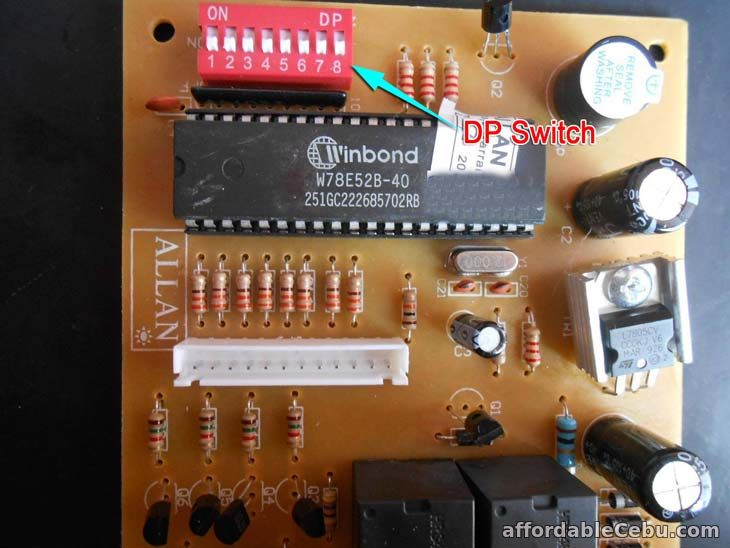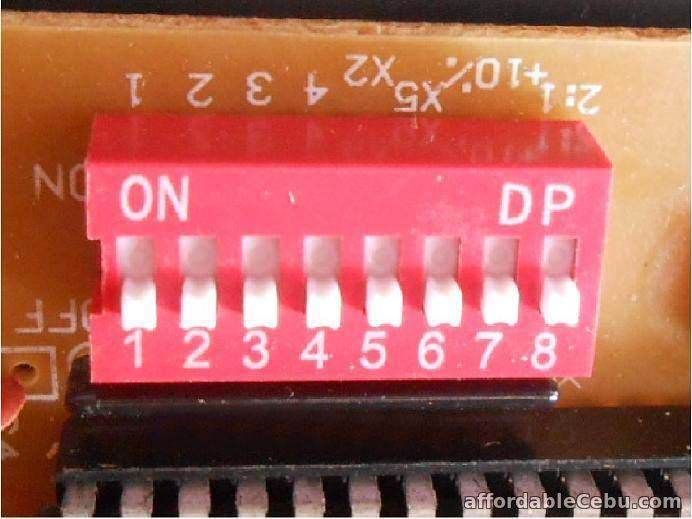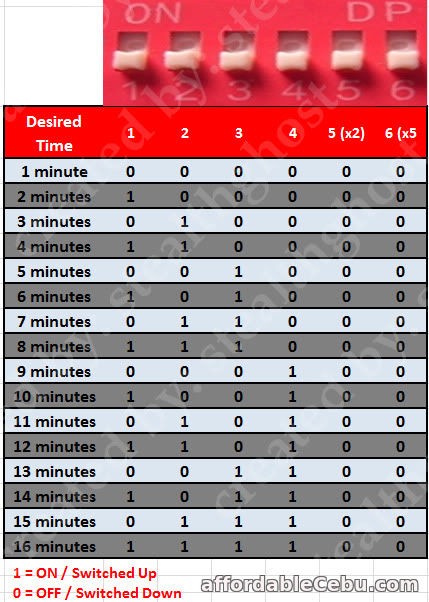Home » Articles » Technology

# How to Configure the TIMER for Pisonet, Washer, Wifi and Other Vending Machines

This is how to set-up or program (configure) the TIMER (specifically the Allan Timer). Set the time (number of minutes) according to the coins inserted.The ALLAN TIMER has a DP switch. This is the red-colored accessory in the circuit board you can see in the picture below:It has 8 tiny switches starting from switch 1, switch 2, and up to switch 8. In the upper-left corner of this DP switch, you can see the word "ON" which means if you switch up the switches, it will turn ON. Likewise, if you switch down the switches, it will turn OFF.

In the picture above, all switches are turned OFF.

Below are the functions of each switch:

• Switch 1 = 1 minute (time value)
• Switch 2 = 2 minutes (time value)
• Switch 3 = 4 minutes (time value)
• Switch 4 = 8 minutes (time value)
• Switch 5 = time value is being multiplied by 2 (x2 multiplier)
• Switch 6 = time value is being multiplied by 5 (x5 multiplier)
• Switch 7 = increase time value by 10% speed
• Switch 8 = 2:1 ratio (2 coins is to 1 count)
The timer's firmware has a default of +1 minute. The 1 minute is added to the total time value.

Take note: The switch 1 to 4 are for the time value and the switches 5 and 6 are for the multiplier of the time value.

Here's how to compute?

Add the time value of all switches turned ON. And add the (+1 minute default time value of the firmware). Then, multiply to the multiplier if the multiplier is turned ON.

Here are some examples below:

• Switch 1 (ON) = 1 minute + 1 (firmware default time value) = 2 minutes
• Switch 2 (ON) = 2 minutes + 1 (default) = 3 minutes
• Switch 2 (ON), Switch 5 (ON) = 2 minutes x 2 (multiplier) = 4 minutes
• Switch 3 (ON) = 4 minutes + 1 (default) = 5 minutes
• Switch 1 (ON), Switch 3 (ON) = 5 minutes + 1 (default) = 6 minutes
• Switch 2 (ON, Switch 3 (ON) = 6 minutes + 1 (default) = 7 minutes
• Switch 1 (ON), Switch 2 (ON), Switch 3 (ON) = 7 minutes + 1 (default) = 8 minutes
• Switch 4 (ON) = 8 minutes + 1 (default) = 9 minutes
• Switch 1 (ON), Switch 4 (ON) = 9 minutes + 1 (default) = 10 minutes
• Switch 2 (ON), Switch 4 (ON) = 10 minutes + 1 (default) = 11 minutes
• Switch 1 (ON), Switch 2 (ON), Switch 4 (ON) = 11 minutes + 1 (default) = 12 minutes
• Switch 3 (ON), Switch 4 (ON) = 12 minutes + 1 (default) = 13 minutes
• Switch 1 (ON), Switch 3 (ON), Switch 4 (ON) = 13 minutes + 1 (default) = 14 minutes
• Switch 2 (ON), Switch 3 (ON), Switch 4 (ON) = 14 minutes + 1 (default) = 15 minutes
• Switch 1 (ON), Switch 2 (ON), Switch 3 (ON), Switch 4 (ON) = 15 minutes + 1 (default) = 16 minutes
• Switch 4 (ON), Switch 5 (ON) = [8 minutes + 1(default)] x 2  = 18 minutes
• Switch 1 (ON), Switch 5 (ON), Switch 6 (ON) = [1 minute + 1 (default)] x 2 x 5 = 20 minutes
• Switch 3 (ON), Switch 6 (ON) = [4 minutes + 1 (default)] x 5 = 25 minutes
To make your TIME CONFIGURATION easier, you can use the table below. Number 1 means turn ON while number 0 means turn OFF:Remember the switch 7 is for the 10% increase time speed. If you want to increase the timer to 10% speed, just turn on the switch 7.

Example:

If you have two (2) TIMER, let's say TIMER 1 has the switch 7 turn ON and other one, TIMER 2, has the switch 7 turn OFF. In a 1 minute or 60 seconds time, TIMER 1 will run out of time faster than the TIMER 2. In a computation, 10% of 60 seconds is 6 seconds. So, TIMER 1 is 6 seconds faster than TIMER 2.

If the time is 30 minutes, TIMER 1 is 3 minutes advance than TIMER 2.

Other PISONET owners uses the Switch 7. They turn it ON. They increases the TIME VALUE but the TIMESPEED will compensate that increase.

And lastly, Switch 8 if for the NUMBER OF COINS to be inserted. If you turn ON the Switch 8, the timer needs 2 coins to be inserted in order to count it as 1 time value. Most of the time, it is turn OFF.

Reference:
This is based on a real experiment that uses the 4-digit ALLAN Timer Board.